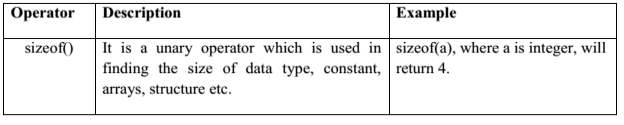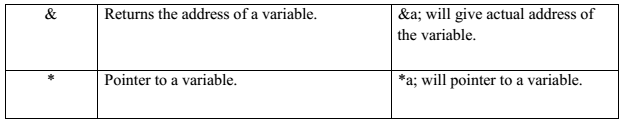In C, ++ and – are called increment and decrement operators respectively. Both of these operators are unary operators, i.e, used on single operand. ++ adds 1 to operand and – subtracts 1 to operand respectively.

For example:

```Let a=5 and b=10
a++; //a becomes 6
a--; //a becomes 5
++a; //a becomes 6
--a; //a becomes 5```

When i++ is used as prefix(like: ++var), ++var will increment the value of var and then return it
but, if ++ is used as postfix(like: var++), operator will return the value of operand first and then
only increment it.

This can be demonstrated by an example:

```#include <stdio.h>
int main()
{
int c=2,d=2;
printf(“%d\n”,c++); //this statement displays 2 then, only c incremented by 1 to 3.
Printf(“%d”,++c); //this statement increments 1 to c then, only c is displayed.
Return 0;
}
Output
2
4```

Conditional Operators (? 🙂

Conditional operators are used in decision making in C programming, i.e, executes different statements according to test condition whether it is either true or false.

Syntax of conditional operators;

conditional_expression?expression1:expression2

If the test condition is true (that is, if its value is non-zero), expression1 is returned and if false expression2 is returned

Let us understand this with the help of a few examples:

```int x, y ;
scanf ( “%d”, &x ) ;
y = ( x> 5 ? 3 : 4 ) ;```

This statement will store 3 in y if x is greater than 5, otherwise it will store 4 in y.
The equivalent if statement will be,

```if ( x > 5 )
y = 3 ;
else
y = 4 ;```

Misc Operators:

There are few other operators supported by c language